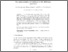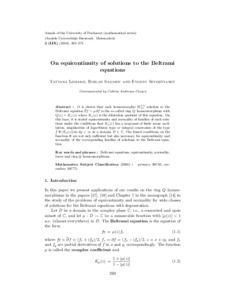# On equicontinuity of solutions to the Beltrami equations

Sevost’yanov, Е. А. and Salіmov, R. R. and Lomaкo, Тatyana (2010) On equicontinuity of solutions to the Beltrami equations. Annals of the University of Bucharest (2). pp. 263-274.Preview
Text
Download (218kB) | Preview

## Abstract

It is shown that each homeomorphic W1;1 loc solution to the Beltrami equation @f = � @f is the so-called ring Q{homeomorphism with Q(z) = K�(z) where K�(z) is the dilatation quotient of this equation. On this base, it is stated equicontinuity and normality of families of such solu- tions under the conditions that K�(z) has a majorant of �nite mean oscil- lRation, singularities of logarithmic type or integral constraints of the type �(K�(z)) dx dy < 1 in a domain D � C: The found conditions on the function � are not only su�cient but also necessary for equicontinuity and normality of the corresponding families of solutions to the Beltrami equa- tion.

Item Type: Article Beltrami equations, equicontinuity, normality, lower and ring Q{homeomorphisms. Q Science > QA Mathematics > Mathematical Analysis Faculty of Physics and Mathematics > Department of Mathematical Analysis Ірина Ігорівна Таргонська 12 Nov 2014 10:01 15 Aug 2015 10:12 http://eprints.zu.edu.ua/id/eprint/13947

### Actions (login required)View Item

### Downloads

Downloads per month over past year

View more statistics